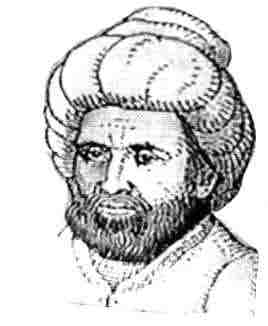## Wednesday, November 15, 2006

### Abu Ja'far Muhammad ibn Musa Al-KhwarizmiUnfortunately, there are many details missing from our knowledge about Abu Ja'far Muhammad ibn Musa Al-Khwarizmi. He was born around 780 AD. It is unclear where he was born. His name Al-Khwarizmi may imply that he was from Khwarizm (Khiva) in Central Asia. Again, this may imply that his family is from Khwarizm but he himself was from Baghdad.

We do know that Al-Kwarizmi became a scholar at the House of Wisdom in Baghdad. His role was to translate books from Greek as well as write original works on mathematics, astronomy, and other topics. The House of Wisdom was an academy that had been set up by Al-Mamun who became caliph of the Arab Empire in 813 AD after defeating his brother. In addition to the House of Wisdom, Al-Mamun set up a great library and and several astronomy observatories. The library at this time was perhaps the most important collection of Greek and Byzantine books since the great library of Alexandria.

We know that Al-Khwarizmi dedicated two of his books to Caliph Al-Mamun. One of these books was on astronomy and the other book was the very famous text on algebra called Hisab al-jabr w'al-muqabala. It is from this book that we get the English work algebra (the Latin translation of the book was Liber algebrae et almucabala). This second book launched the rise of algebra in its ascendency over the geometry of the Greeks. Another of his books on arithmetic popularized what became known as Arabic numerals.

The long title of the book on algebra refers to two important operations. al-jabr ("completion") and al-muqabala ("balancing"). The book systematizes linear and quadratic equations and focuses on numbers (as opposed to geometric shapes) which include units (a number), roots (x), and squares (x2).

The book is written almost completely in words. Even numbers are written as words. Although Al-Khwarizmi popularized the Arabic numerals in another of his books, he does not use them here. He categorizes quadratic equations into six types:
• "squares" equal "roots" (ax2 = bx)
• "squares" equal number (ax2 = c)
• "roots" equal number (bx = c)
• "squares" and "root" equal number (ax2 + bx = c)
• "squares" and number equal "roots" (ax2 + c = bx)
• "roots" and number equal "square" (bx + c = ax2)
He then presents algebraic and geometric methods for solving each of these types.

Interestingly, his book does not include a the general solution for quadratic equations even though, it is implied in the solution for each of the problems in the book. This would be first published in 1145 by Abraham bar Hiyya Ha-Nasi.

Al-Khwarizmi's book on arithmetic was called Al-Khwarizmi on the Hindu Art of Reckoning. Unfortunately, all Arabic versions of this book have been lost. The only extent book is a Latin translation.

This book on arithmetic has given us the modern word "algorithm" and it is the place where the Hindu numerals are introduced to the West and the place where 0 is used as a number. This book consists of a systematization of the Indian numerals.

Al-Khwarizmi wrote many other books including books on astronomy, geography, calendars, sun dials, and many other topics. Unfortunately, it is very difficult to know how much of this content was original and how much was taken from other sources.

What is undeniable is the great importance of Al-Khwarizmi's work and the impact it had on the rise of algebra.

One scholar has written (see the MacTutor reference):
In the foremost rank of mathematicians of all time stands Al-Khwarizmi. He composed the oldest works on arithmetic and algebra. They were the principal source of mathematical knowledge for centuries to come in the East and the West. The work on arithmetic first introduced the Hindu numbers to Europe, as the very name algorism signifies; and the work on algebra ... gave the name to this important branch of mathematics in the European world...

References